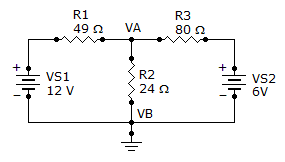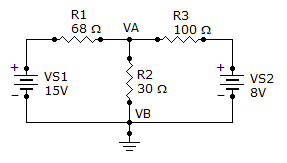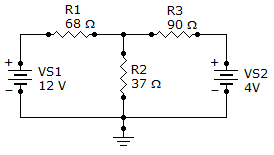# Electrical Engineering - Branch, Loop and Node Analyses

Exercise : Branch, Loop and Node Analyses - General Questions
1.
Find the node voltage VA.6 V
12 V
4.25 V
3 V
Explanation:
No answer description is available. Let's discuss.

2.
What is the current through R2?3.19 A
319 mA
1.73 A
173 mA
Explanation:
No answer description is available. Let's discuss.

3.
Find branch current IR2.5.4 mA
–5.4 mA
113.0 mA
119.6 mA
Explanation:
No answer description is available. Let's discuss.

4.
Find the node voltage VA.518 mV
5.18 V
9.56 V
956 mV
Explanation:
Reason: The voltage drop through R2 is 5.18v.

5.

Find I2.

4I1 + 4I2 = 2
6I1 + 7I2 = 4
1 A
–1 A
100 mA
–100 mA